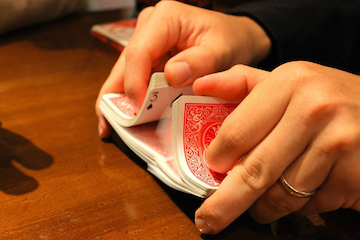# Where are the aces?

Probability Level 2A standard deck of 52 cards is shuffled randomly, and then the cards are flipped over one-by-one. If the ace of spades is card number $S$ and the ace of hearts is card number $H$, what is the expected value of $S+H?$

×